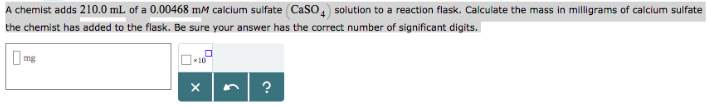# Problem: A chemist adds 210.0 mL of a 0.00468 mM calcium sulfate (CaSO4) solution to a reaction flask. Calculate the mass in milligrams of calcium sulfate the chemist has added to the flask. Be sure your answer has the correct number of significant digits.

###### FREE Expert Solution
80% (487 ratings)###### Problem Details

A chemist adds 210.0 mL of a 0.00468 mM calcium sulfate (CaSO4) solution to a reaction flask. Calculate the mass in milligrams of calcium sulfate the chemist has added to the flask. Be sure your answer has the correct number of significant digits.